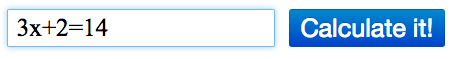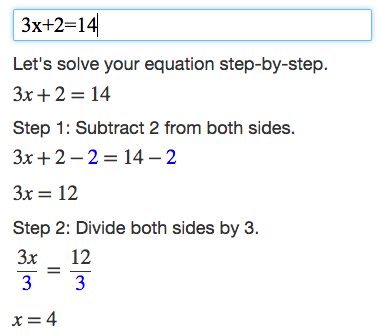# Algebra Calculator

Step-by-step calculator that understands algebra.

## Quick Tutorial

This is a quick tutorial for the Algebra Calculator, a step-by-step calculator for algebra.

### How to Use the Calculator

To use the calculator, you enter the math problem that you want to calculate into the text box.For example, if you enter the equation 3x+2=14 into the text box, the calculator will print a step-by-step explanation of how to solve 3x+2=14.### Examples

Trying the examples on the Examples page is the quickest way to learn how to use the calculator.

### Math Symbols

If you would like to create your own math expressions, here are some symbols that the calculator understands: